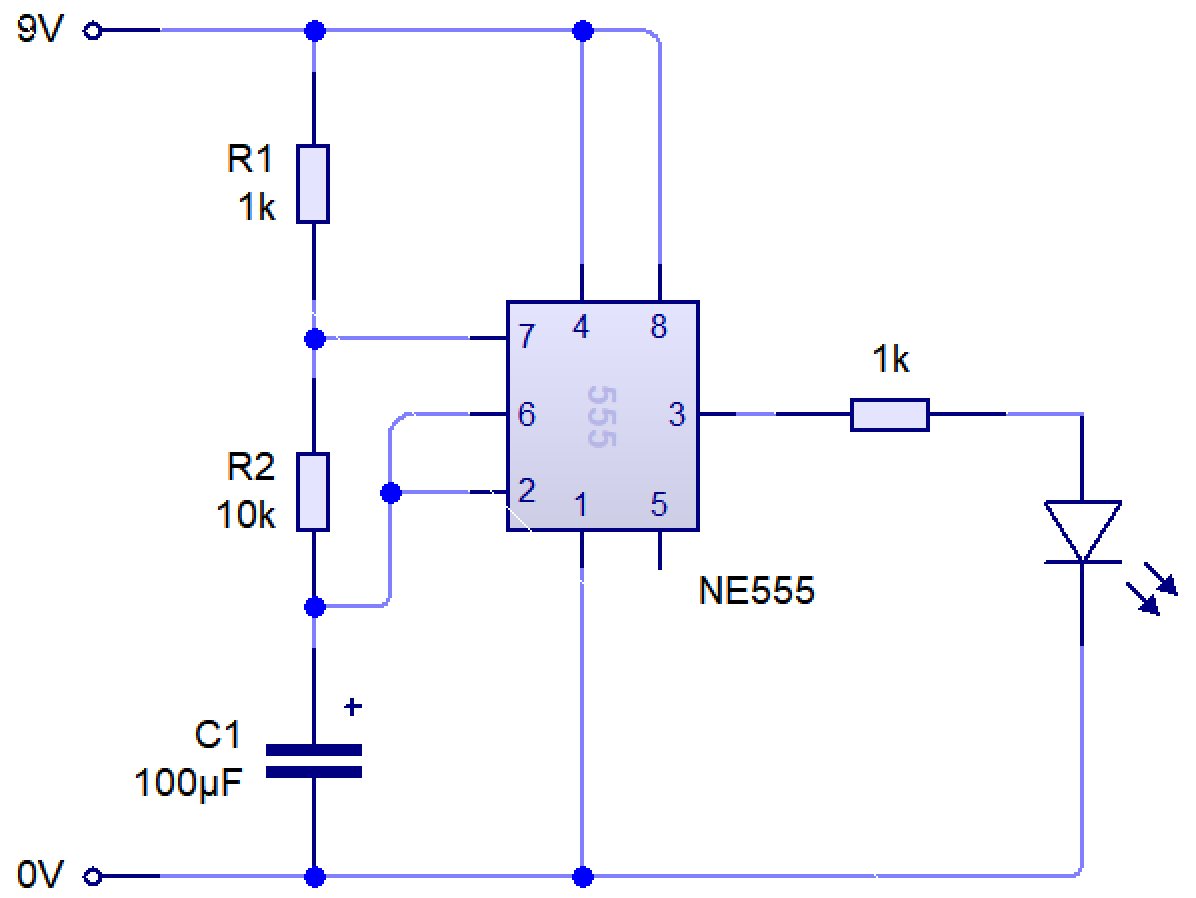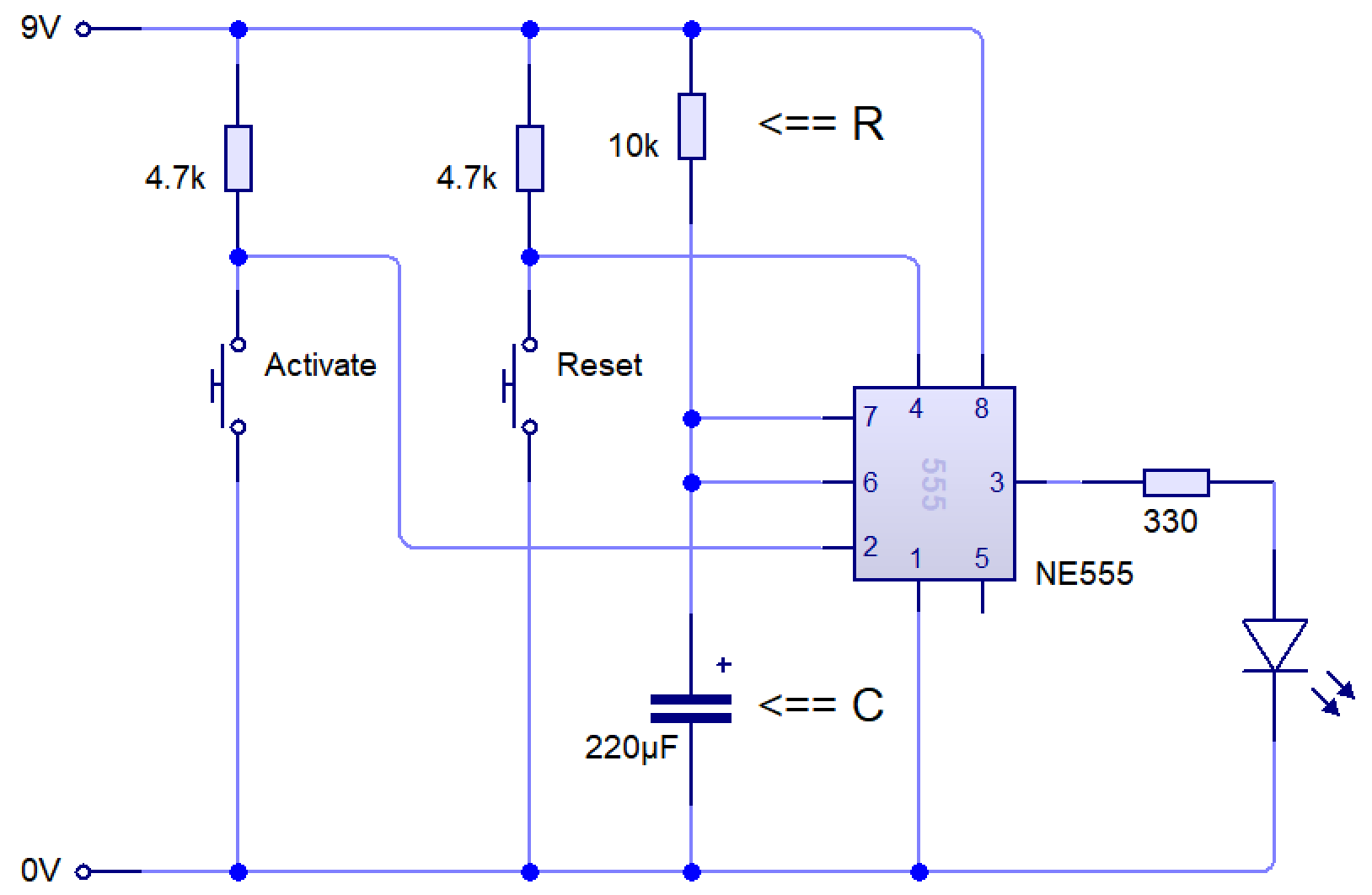# Egg Timer

### Learn It

• Boiling an egg requires perfect timing.
• There are many ways to create a timing circuit, but one of the simplest ways is to use a 555 timercircuit.
• The 555 timer circuit relies on a basic electronic timing circuit which uses resistors and capacitors to use time in different ways.
• A capacitor takes time to charge. We can use this, along with a resistor to create different time delays.
• It's output pin (3) can be made to change its behaviour with these two simple components.
• This is the basis of this project.

### Learn It

• A 555 timer can be made to work in a few different ways by changing the circuit slightly.• The circuit diagram above is called `astable`. You can see it running live below.

• The term 'astable' means that the output pin has no stable state; it will constantly flip between being on and off.
• We can calculate how long it stays on (high) and off (low) for by using a few formulae.
• We can calculate how long the output will stay on (high) for in seconds, with:• We can see how long the output will be low for (in seconds) with:• Finally, we can calculate the frequency (number of on/off cycles per second, measured in Hertz) with:• In the example above, resistor 1 has a value of 1k Ohms. 1k is shorthand for 1000, so R1 = 1000
• Resistor 2 has the value 10k, so R2 = 10000
• The capactor (C) has the value 100 μF. μF is shorthand for 'microfarads'.
• You may already know from science that putting a μ before a measurement means that what we are actually writing is that number x10-6.
• So C = 100 μF = 100 x 10-6 = 0.000100 = 0.0001
• We can now calculate the characteristics of our circuit.
• Time High = 0.7 x (1000 + 10000) x 0.0001
• Time High = 0.77s
• Time Low = 0.7 x 10000 x 0.0001
• Time Low = 0.7s
• Frequency = 1.44 / ((1000 + (2x10000) x 0.0001))
• Frequency = 1.44 / ((1000 + 20000) x 0.0001)
• Frequency = 1.44 / (21000 x 0.0001)
• Frequency = 1.44 / 2.1 = 0.69Hz
• These are handy for all sorts of applications where you want something to flip between on and off constantly. E.g.
• A strobe lighting system for a theatre; the light is flipped on and off several times per second.
• A sound generator pulsing a speaker (555 timers can pulse thousands of times a second).
• A daily automatic fish food dispenser (they can also wait up to about three days between going from off to on).
• A speed controller for a model aeroplane (pulsing a motor on and off swiftly will make it run more slowly).
• A set of lights on top of an emergency response vehicle.
• The Year 7 555 piano. Different resistors make the pitch different. It doesn't have to always be an LED.

### Learn It

• Another way is called 'monostable', as the circuit has one stable state: Being turned off.• In this setup, the output pin (3) stays off until an input (e.g. a switch) is triggered.
• Once triggered, the output is turned on for a set time, then goes back to being off again.
• We can calculate the time delay using a simple formula, T = 1.1 x R x C
• In the example above, R is 10k (so R = 10000)
• C is 220μF. Written in Farads, this is 220 x 10-6 = 0.000220.
• T = 1.1 x 10000 x 0.00022
• T = 2.42 seconds.
• These are handy for all sorts of applications where you want something to happen for a set period of time. E.g.
• A patio heater which is turned on for 2 minutes when a switch is pressed.
• A security door for a block of flats, where the door lock is released for 5 seconds when the owner 'buzzes you in'.
• A door bell, where the bell rings for 3 seconds when someone pushes the bell switch.
• A hand drier, where the heater and fan are energised for 15 seconds when someone waves their hand under the sensor.
• An outdoor security light, where a floodlight is energised for a minute when someone walks past.

### Try It

• Try building the `Monostable circuit` above using circuit wizard.
• Change the value of `R` and see how it affects the speed at which the LED flashes.